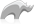##### [ staticarray->sub ]

Method

The [staticarray->sub] method returns a staticarray containing elements from the original array.

The method requires one integer parameter which is the index of the element to be returned from the original array. Optionally it may take a second integer parameter which is the number of objects to return (including the first) from the original array. If this parameter is omitted [staticarray->sub] returns all elements from the specified index to the end of the original array.

Note: This method will error if, either the index parameter or the sum of the index parameter and the length parameter is greater than the size of the original array.

• Syntax
``````staticarray->sub( index )

staticarray->sub( index, length )``````
• Beginner

### To produce a staticarray which is a subset of another array

Use the [staticarray->sub] method.

In the following example we will create two new staticarrays from a staticarray of  days of the week. The first will be an array of days after Wednesday called \$endOfWeek, and the second will be an array of weekdays called \$weekdays.

Code

``````var( DaysOfWeek = staticarray('Sun', 'Mon', 'Tue', 'Wed', 'Thu', 'Fri', 'Sat') )

var( endOfWeek = \$DaysOfWeek->sub(5) )
var( weekdays = \$DaysOfWeek->sub(2,5) )

'endOfWeek = '+\$endOfWeek+'\n'
'weekdays = '+\$weekdays``````

Result

```endOfWeek = staticarray(Thu, Fri, Sat)
weekdays = staticarray(Mon, Tue, Wed, Thu, Fri)```Please note that periodically LassoSoft will go through the notes and may incorporate information from them into the documentation. Any submission here gives LassoSoft a non-exclusive license and will be made available in various formats to the Lasso community.